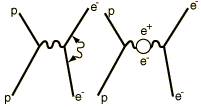# The Lamb Shift

According to the hydrogen Schrodinger equation solution, the energy levels of the hydrogen electron should depend only on the principal quantum number n. In 1951, Willis Lamb discovered that this was not so - that the 2p(1/2) state is slightly lower than the 2s(1/2) state resulting in a slight shift of the corresponding spectral line (the Lamb shift).

 It might seem that such a tiny effect would be deemed insignificant, but in this case that shift probed the depths of our understanding of electromagnetic theory.At the heart of the process is the exchange force by which charges interact by the exchange of photons (the exchange force model of the electromagnetic force). There can be a self-interaction of the electron by exchange of a photon as sketched in the Feynman diagram at left. This "smears out" the electron position over a range of about 0.1 fermi (Bohr radius = 52,900 fermis). This causes the electron spin g-factor to be slightly different from 2. There is also a slight weakening of the force on the electron when it is very close to the nucleus, causing the 2s electron (which has penetration all the way to the nucleus) to be slightly higher in energy than the 2p(1/2) electron.

When we say the that the penetration of the 2s electron closer to the nucleus leads to an energy higher than that of the 2p, this sounds directly contradictory to the situation with multi-electron atoms. There the penetration of the 2s closer to the nucleus means that it has penetrated inside the 1s electrons and therefore feels a stronger attraction to the positive nucleus, leading to a lower energy level (it takes more energy to remove the 2s from the atom than the 2p). But in the case of the hydrogen atom, there is only one electron, so there is none of the shielding from inner electrons when it is in the 2s or 2p excited states. The effect on the energy levels has an entirely different origin, modeled by quantum electrodynamics. In the absence of this effect, the 2s and 2p would have identical energies since there is no shielding by the presence of other electrons. The "self-interaction" of the electron when it is near the proton causes the effective "smearing" of the electron charge so that its attraction to the proton is slightly weaker than it otherwise would have been. This means it has encountered an interaction which makes it slightly less tightly bound than a 2p electron, hence higher in energy.Active illustration: click for more detail

 Microwave measurement of the Lamb shift. Significance of the Lamb shift
 Saturation spectroscopy measurement of the Lamb shift.
Index

Schrodinger equation concepts

Hydrogen concepts

Reference
Rohlf
Sec 8-9.

Lamb Shift Washington.edu

Lamb Shift wiki

 HyperPhysics***** Quantum Physics R Nave
Go Back

# Measurement of the Lamb Shift

While the Lamb shift is extremely small and difficult to measure as a splitting in the optical or UV spectral lines, it is possible to make use of transitions directly between the sublevels by going to other regions of the electromagnetic spectrum. Willis Lamb and Robert Retherford made their measurements of the shift in the microwave region. They formed a beam of hydrogen atoms in the 2s(1/2) state. These atoms could not directly take the transition to the 1s(1/2) state because of the selection rule which requires the orbital angular momentum to change by 1 unit in a transition. Putting the atoms in a magnetic field to split the levels by the Zeeman effect, he exposed the atoms to microwave radiation at 2395 MHz (not too far from the ordinary microwave oven frequency of 2450 MHz).Then they varied the magnetic field until that frequency produced transitions from the 2p(1/2) to 2p(3/2) levels. They could then measure the allowed transition from the 2p(3/2) to the 1s(1/2) state. They used the results to determine that the zero-magnetic field splitting of these levels correspond to 1057 MHz. By the Planck relationship, this told then that the energy separation was 4.372x 10-6 eV.

A large Doppler broadening was avoided in the Lamb-Retherford experiment by using the microwave transitions where the Doppler shift was small. Using the 2.395 GHz microwaves for the experiment gives a Doppler shift of only about 20 kHz which did not hinder the experiment. The saturation spectroscopy experiment also avoided the Doppler broadening problem.

 Saturation spectroscopy measurement of the Lamb shift.
Index

Schrodinger equation concepts

Reference
Rohlf
Sec 8-9.

Lamb Shift wiki

 HyperPhysics***** Quantum Physics R Nave
Go Back

# Significance of the Lamb Shift

When the Lamb shift was experimentally determined, it provided a high precision verification of theoretical calculations made with the quantum theory of electrodynamics. These calculations predicted that electrons continually exchanged photons, this being the mechanism by which the electromagnetic force acted. The effect of the continuous emission and absorption of photons on the electron g-factor could be calculated with great precision.

The tiny Lamb shift, measured with great precision, agreed to many decimal places with the calculated result from quantum electrodynamics. The measured precision gives us the electron spin g-factor as

g=2.002319304386
Index

Schrodinger equation concepts

Reference
Rohlf
Sec 8-9.

Richtmyer, et al.,
Sec 18.4.

 HyperPhysics***** Quantum Physics R Nave
Go Back